## Names to Formulas 2 - Questions

The Names to Formulas 2 Concept Builder is comprised of 42 questions. The questions are divided into 14 different Question Groups and spread across three difficulty levels. Questions in the same group are rather similar to one another. The Concept Builder is coded to select at random a question from each group until a student is successful with that group of questions.

There are three difficulty levels that can be engaged in through the Concept Builder. Those three activities are differentiated as follows:

• Apprentice Difficulty Level: Question Groups 1-4 ... Compounds include molecular compounds composed of two non-metal elements. Students must identify formulas that are inconsistent with the given name.
• Master Difficulty Level: Question Groups 5-8 ... Compounds include non-binary ionic compounds composed of a polyatomic ion. Students must identify formulas that are inconsistent with the given name.
• Wizard Difficulty Level: Question Groups 9-14 ... Each question includes a mix of binary molecular and ionic compounds and non-binary compounds containing polyatomic ions.

The questions from each group are shown below. Teachers are encouraged to view the questions in order to judge which difficulty levels are most appropriate for their classes. We recommend providing students two or more options.

The Physics Classroom grants teachers and other users the right to print these questions for private use. Users are also granted the right to copy the text and modify it for their own use. However, this document should not be uploaded to other servers for distribution to and/or display by others. The Physics Classroom website should remain the only website or server from which the document is distributed or displayed. We also provide a PDF that teachers can use under the same conditions. We have included a link to the PDF near the bottom of this page.

#### Names to Formulas 2

Apprentice Difficulty Level
Question Group 1
Question 1
In Chemistry, there are specific rules for writing the formulas of compounds having a given name. One or more of these formulas are breaking the rules. Select all the rule breakers.Question 2
In Chemistry, there are specific rules for writing the formulas of compounds having a given name. One or more of these formulas are breaking the rules. Select all the rule breakers.Question 3
In Chemistry, there are specific rules for writing the formulas of compounds having a given name. One or more of these formulas are breaking the rules. Select all the rule breakers.Question Group 2
Question 4
In Chemistry, there are specific rules for writing the formulas of compounds having a given name. One or more of these formulas are breaking the rules. Select all the rule breakers.Question 5
In Chemistry, there are specific rules for writing the formulas of compounds having a given name. One or more of these formulas are breaking the rules. Select all the rule breakers.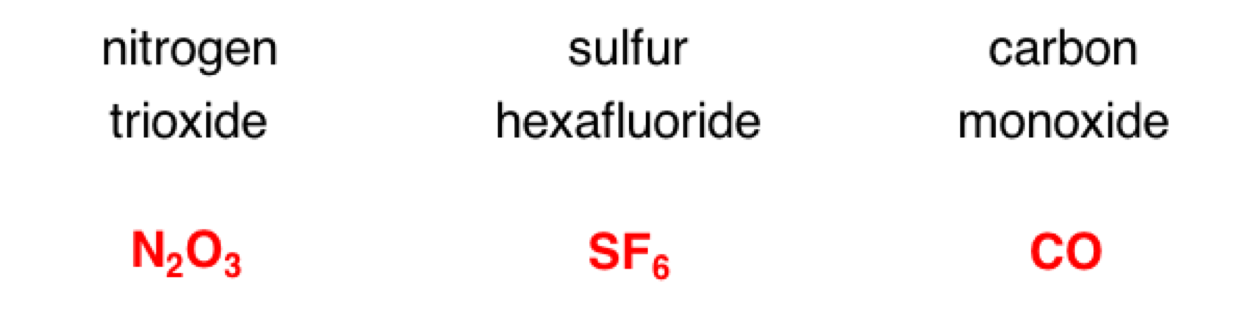Question 3
In Chemist6y, there are specific rules for writing the formulas of compounds having a given name. One or more of these formulas are breaking the rules. Select all the rule breakers.Question Group 3
Question 7
In Chemistry, there are specific rules for writing the formulas of compounds having a given name. One or more of these formulas are breaking the rules. Select all the rule breakers.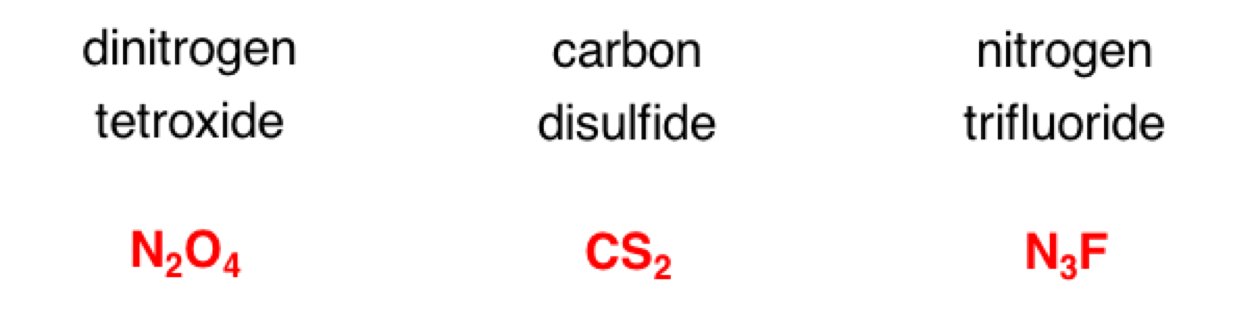Question 8
In Chemistry, there are specific rules for writing the formulas of compounds having a given name. One or more of these formulas are breaking the rules. Select all the rule breakers.Question 9
In Chemistry, there are specific rules for writing the formulas of compounds having a given name. One or more of these formulas are breaking the rules. Select all the rule breakers.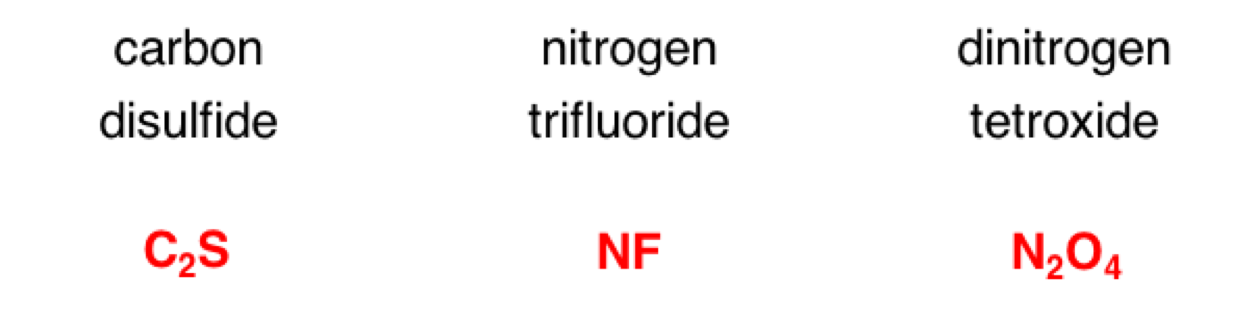Question Group 4
Question 10
In Chemistry, there are specific rules for writing the formulas of compounds having a given name. One or more of these formulas are breaking the rules. Select all the rule breakers.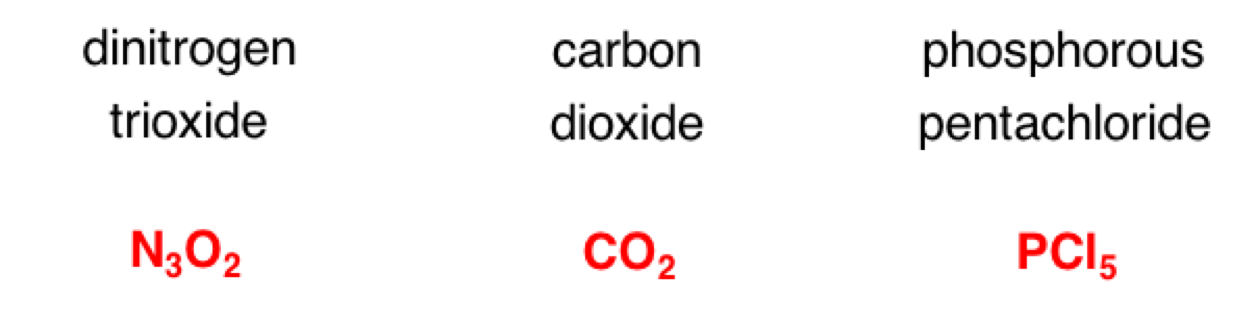Question 11
In Chemistry, there are specific rules for writing the formulas of compounds having a given name. One or more of these formulas are breaking the rules. Select all the rule breakers.Question 12
In Chemistry, there are specific rules for writing the formulas of compounds having a given name. One or more of these formulas are breaking the rules. Select all the rule breakers.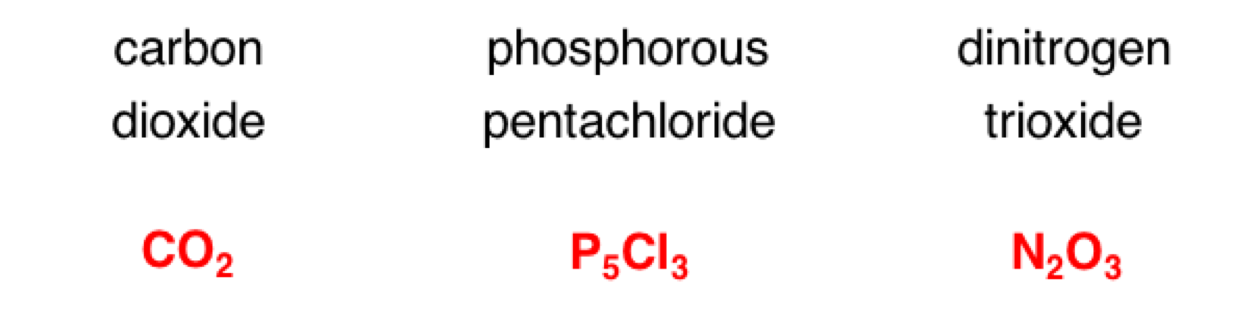Master Difficulty Level
Question Group 5
Question 13
In Chemistry, there are specific rules for writing the formulas of compounds having a given name. One or more of these formulas are breaking the rules. Select all the rule breakers.Question 14
In Chemistry, there are specific rules for writing the formulas of compounds having a given name. One or more of these formulas are breaking the rules. Select all the rule breakers.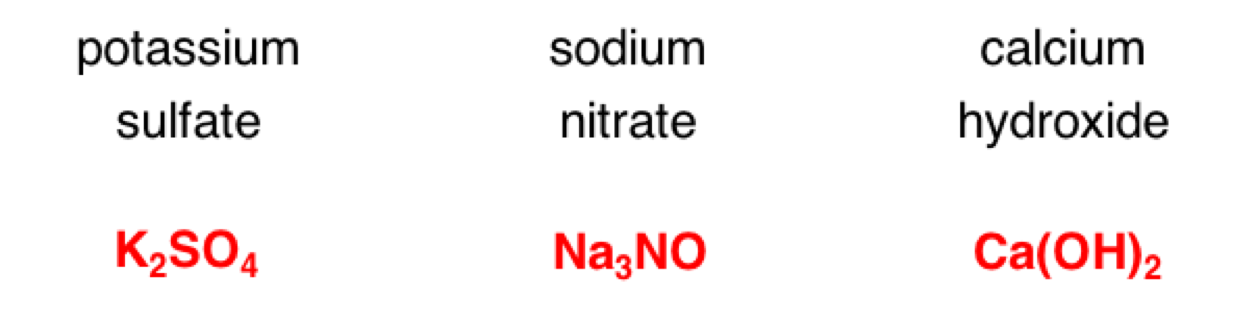Question 15
In Chemistry, there are specific rules for writing the formulas of compounds having a given name. One or more of these formulas are breaking the rules. Select all the rule breakers.Question Group 6
Question 16
In Chemistry, there are specific rules for writing the formulas of compounds having a given name. One or more of these formulas are breaking the rules. Select all the rule breakers.Question 17
In Chemistry, there are specific rules for writing the formulas of compounds having a given name. One or more of these formulas are breaking the rules. Select all the rule breakers.Question 18
In Chemist6y, there are specific rules for writing the formulas of compounds having a given name. One or more of these formulas are breaking the rules. Select all the rule breakers.Question Group 7
Question 19
In Chemistry, there are specific rules for writing the formulas of compounds having a given name. One or more of these formulas are breaking the rules. Select all the rule breakers.Question 20
In Chemistry, there are specific rules for writing the formulas of compounds having a given name. One or more of these formulas are breaking the rules. Select all the rule breakers.Question 21
In Chemistry, there are specific rules for writing the formulas of compounds having a given name. One or more of these formulas are breaking the rules. Select all the rule breakers.Question Group 8
Question 22
In Chemistry, there are specific rules for writing the formulas of compounds having a given name. One or more of these formulas are breaking the rules. Select all the rule breakers.Question 23
In Chemistry, there are specific rules for writing the formulas of compounds having a given name. One or more of these formulas are breaking the rules. Select all the rule breakers.Question 24
In Chemistry, there are specific rules for writing the formulas of compounds having a given name. One or more of these formulas are breaking the rules. Select all the rule breakers.Wizard Difficulty Level
Question Group 9
Question 25
In Chemistry, there are specific rules for writing the formulas of compounds having a given name. One or more of these formulas are breaking the rules. Select all the rule breakers.Question 26
In Chemistry, there are specific rules for writing the formulas of compounds having a given name. One or more of these formulas are breaking the rules. Select all the rule breakers.Question 27
In Chemistry, there are specific rules for writing the formulas of compounds having a given name. One or more of these formulas are breaking the rules. Select all the rule breakers.Question Group 10
Question 28
In Chemistry, there are specific rules for writing the formulas of compounds having a given name. One or more of these formulas are breaking the rules. Select all the rule breakers.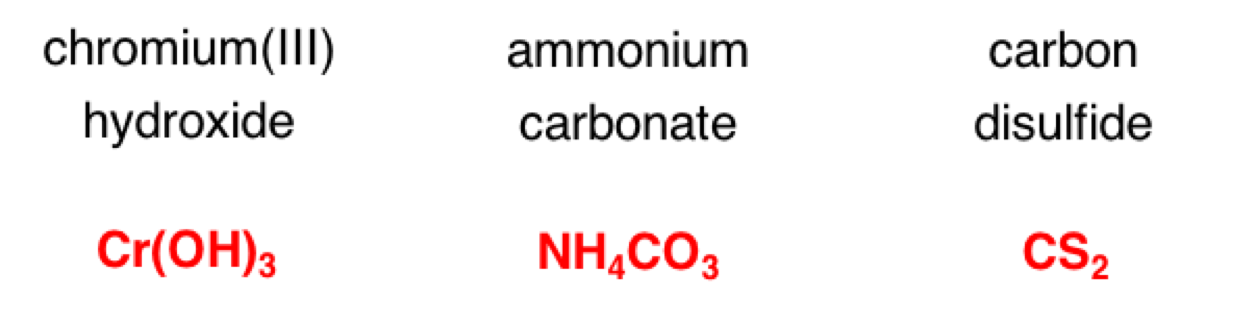Question 29
In Chemistry, there are specific rules for writing the formulas of compounds having a given name. One or more of these formulas are breaking the rules. Select all the rule breakers.Question 30
In Chemist6y, there are specific rules for writing the formulas of compounds having a given name. One or more of these formulas are breaking the rules. Select all the rule breakers.Question Group 11
Question 31
In Chemistry, there are specific rules for writing the formulas of compounds having a given name. One or more of these formulas are breaking the rules. Select all the rule breakers.Question 32
In Chemistry, there are specific rules for writing the formulas of compounds having a given name. One or more of these formulas are breaking the rules. Select all the rule breakers.Question 33
In Chemistry, there are specific rules for writing the formulas of compounds having a given name. One or more of these formulas are breaking the rules. Select all the rule breakers.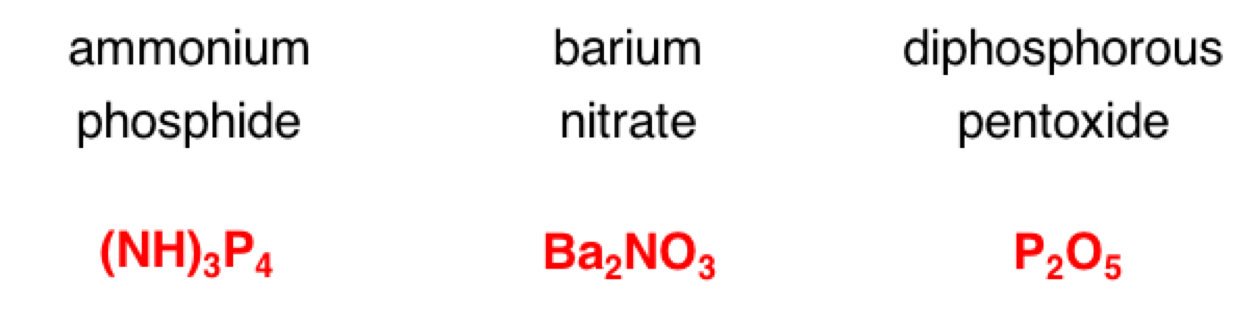Question Group 12
Question 34
In Chemistry, there are specific rules for writing the formulas of compounds having a given name. One or more of these formulas are breaking the rules. Select all the rule breakers.Question 35
In Chemistry, there are specific rules for writing the formulas of compounds having a given name. One or more of these formulas are breaking the rules. Select all the rule breakers.Question 36
In Chemistry, there are specific rules for writing the formulas of compounds having a given name. One or more of these formulas are breaking the rules. Select all the rule breakers.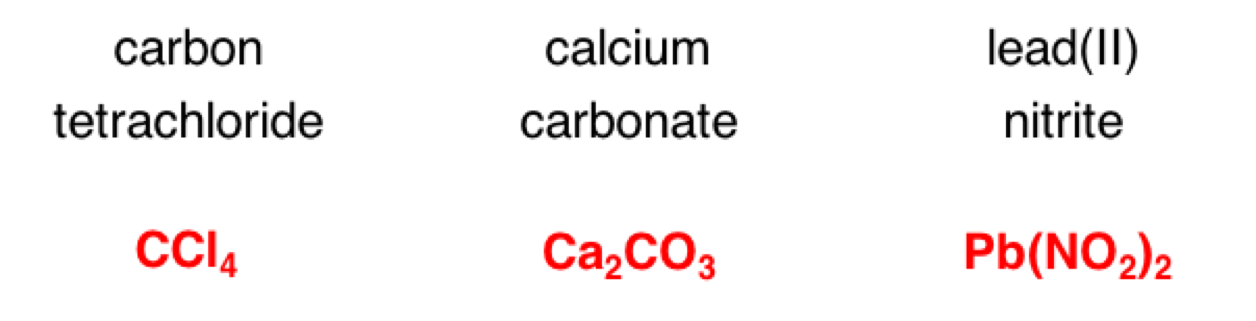Question Group 13
Question 37
In Chemistry, there are specific rules for writing the formulas of compounds having a given name. One or more of these formulas are breaking the rules. Select all the rule breakers.Question 38
In Chemistry, there are specific rules for writing the formulas of compounds having a given name. One or more of these formulas are breaking the rules. Select all the rule breakers.Question 39
In Chemistry, there are specific rules for writing the formulas of compounds having a given name. One or more of these formulas are breaking the rules. Select all the rule breakers.Question Group 14
Question 40
In Chemistry, there are specific rules for writing the formulas of compounds having a given name. One or more of these formulas are breaking the rules. Select all the rule breakers.Question 41
In Chemistry, there are specific rules for writing the formulas of compounds having a given name. One or more of these formulas are breaking the rules. Select all the rule breakers.Question 42
In Chemistry, there are specific rules for writing the formulas of compounds having a given name. One or more of these formulas are breaking the rules. Select all the rule breakers.